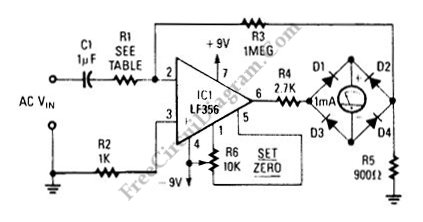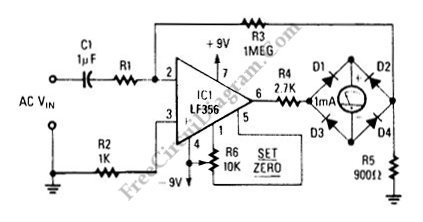# LF356 4-Ranges Ac MillivoltmeterThis is a 4-range AC millivolt meter circuit. This circuit is used to measure a AC voltage in millivolt range. This circuit has frequency response of a few Hz to about 50 kHz. The non-linearity of the rectifier circuit is eliminated by placing the rectifier inside the op amp feedback path. This circuit read the input signal’s full-wave rectified average. Here is the schematic diagram of the  circuit:This circuit has 4 range, they are 1V, 100mV, 10mV, and 1mV. If the voltage full scale is 1V, the R1 value is 1M. Using the 100K for R1, the full scale will be 100mV. R1 is 10K for 10mV voltage full scale and 1K for 1mV.